Next: Phase delay and group Up: Transforms Previous: Z-TRANSFORM TO FOURIER TRANSFORM

# THE FAST FOURIER TRANSFORM

When we write the expression(23)
we have both a time function and its Fourier transform. If we plot the coefficients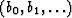, we plot the time function. If we evaluate and plot (23) at numerous real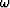,then we have plotted the transform. (Note that for real, Z is of unit magnitude; i.e., on the unit circle.) Sinceis a continuous variable and everything in a computer is finite, how do we select a finite number of valuesfor plotting? The usual choice is to take evenly spaced frequencies. The lowest frequency can be zero. [Note.] A frequency as high as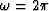[Note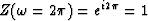also] need not be considered, since (23) gives the same value for it as for zero frequency. Choosing uniformly spaced frequencies between these limits we have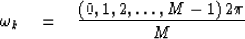(24)
where M is some integer. Now let us abbreviate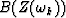as Bk.

For the special case of an N-point time function where N = 4, (23) may be expressed by the matrix multiplication(25)
where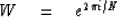(26)
It is not essential to choose N = M as we have done in (25), but it is a convenience. There is no loss of generality because one may always append zeros to a time function before inserting it into (25). A convenience of the choice N = M is that the matrix in (25) will then be square and there will be an exact inverse. In fact, the inverse to (25) may be easily shown to be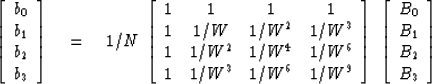(27)

Since 1/W is the complex conjugate of W, the matrices of (25) and (27) are just complex conjugates of one another. In fact, one observes no fundamental mathematical difference between time functions and frequency functions. This duality'' would be even more complete if we had used a scale factor of N-1/2 in each of (25) and (27) rather than 1 in (25) and N-1 in (27). Note also that time functions and frequency functions could be interchanged in the mnemonic table describing symmetries. In fact, our earlier observation that the product of two frequency functions amounts to a convolution of two time functions corresponds to the convolution of the corresponding two frequency functions. We will not provide'' this duality as it is standard fare in both mathematics and systems theory books. However we will occasionally call upon the reader to realize that in any theorem the meanings of time'' and frequency'' may be interchanged.

In making a plot of the transform Bk for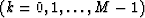,the frequency axis ranges as.It is often more natural to display the interval.Since the transform is periodic with period,values of Bk on the interval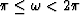may simply be moved to the intervalfor display.

Thus, for N = 8 one might plot successively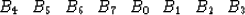(28)
corresponding to values ofequal to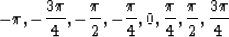(29)
One advantage of this display interval is that for continuous time series which are sampled sufficiently densely in time the transform values Bk get small on both ends. If the time series is real, the real part of Bk has even symmetry about B0; the imaginary part has odd symmetry about B0. Then, one need not bother to display half the values. Choice of an odd value of N would enable us to put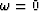exactly in the middle of the interval, but the reader will soon see why we stick to an even number of data points.

The matrix times vector operation in (25) requires N2 multiplications and additions. The rest of this section describes a trick method, called the fast Fourier transform, of accomplishing the matrix multiplication in N log2 N multiplications and additions. Since, for example, log2 1024 is 10, this is a tremendous saving in effort.

A basic building block in the fast Fourier transform is called doubling. Given a series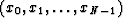and its sampled Fourier transform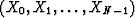and another series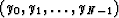,one finds the transform of the interlaced double-length series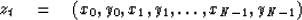(30)
The process of doubling is used many times during the process of computing a fast Fourier transform. As the word doubling might suggest, it will be convenient to suppose that N is an integer formed by raising 2 to some integer power. Suppose N = 8 = 23. We begin by dividing our eight-point series of one point each. The Fourier transform of each of the one-point series is just the point. Next, we use doubling four times to get the transforms of the four different two point series (x0, x4), (x1, x5), (x2, x6), and (x3, x7). We use doubling twice more to get the transforms of the two different four point series (x0, x2, x4, x6) and (x1, x3, x5, x7). Finally, we use doubling once more to get the transform of the original eight-point series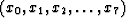.

It remains to look into the details of the doubling process.

Let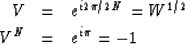(31) (32)
The transforms of two N-point series are by definition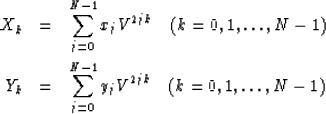(33) (34)
The transform of the interlaced seriesis by definition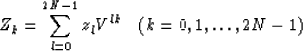(35)

To make Zk from Xk and Yk we require two separate formulas: one for k = 0, 1, ..., N -1, and the other for k = N, N + 1, ..., 2N - 1.

First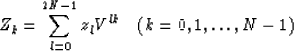We split the sum into two parts, noting that xj multiplies even powers of V and yj multiplies odd powers.(36) (37)
We obtain the last half of the Zk by(38) (39) (40)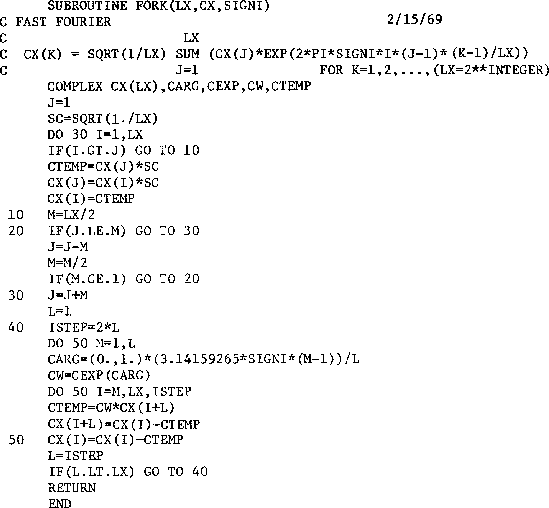1-8
Figure 8
A program to do fast Fourier transform. Modified from Brenner. Calling this program twice returns the original data. SIGNI should be +1. on one call and -1. on the other. LX must be a power of 2.Next: Phase delay and group Up: Transforms Previous: Z-TRANSFORM TO FOURIER TRANSFORM
Stanford Exploration Project
10/30/1997